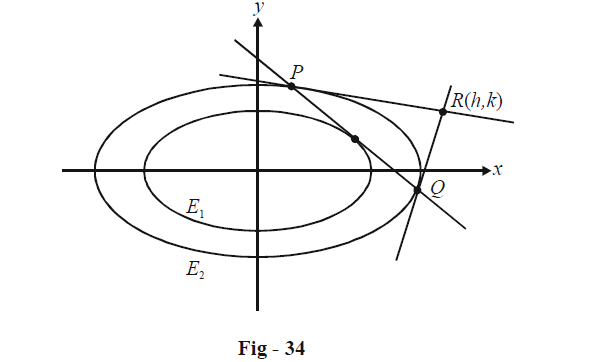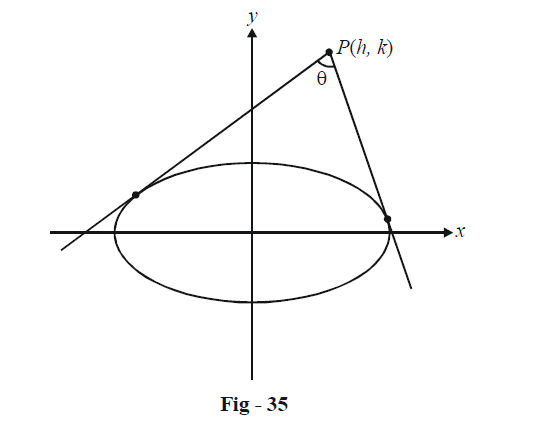# Examples On Tangents And Chords Of Ellipses Set-2

Go back to  'Ellipse'

Example – 28

A tangent drawn to the ellipse \begin{align}{E_1}:\frac{{{x^2}}}{{{a^2}}} + \frac{{{y^2}}}{{{b^2}}} = 1\end{align} intersects the ellipse \begin{align}{E_2}:\frac{{{x^2}}}{a} + \frac{{{y^2}}}{b} = a + b\end{align} at P and Q. Prove that the tangents drawn to E2 at P and Q intersect at right angles.

Solution: Let the point of intersection be $$R(h,\,\,k)$$PQ is the chord of contact for the tangents drawn from $$R(h,\,\,k)$$ to E2. Thus, the equation of PQ is

\begin{align}&T(h,\,\,k) = 0\\& \Rightarrow \quad \frac{{hx}}{a} + \frac{{ky}}{b} = a + b\\& \Rightarrow \quad y = \left( {\frac{{ - bh}}{{ak}}} \right)x + \frac{b}{k}(a + b)\end{align}

PQ touches the inner ellipse E1 if the condition for tangency for ellipses $$({c^2} = {a^2}{m^2} + {b^2})$$ is satisfied. Thus,

\begin{align}&\frac{{{b^2}}}{{{k^2}}}{(a + b)^2} = {a^2}\left( {\frac{{{b^2}{h^2}}}{{{a^2}{k^2}}}} \right) + {b^2}\\ &\Rightarrow \quad {h^2} + {k^2} = {(a + b)^2}\quad\quad\quad...\left( 1 \right)\end{align}

By Example -17, any point $$(h',\,\,k')$$ lying on the director circle of E2 must satisfy

\begin{align}&{{h'}^2} + {{k'}^2}\; = a(a + b) + b(a + b)\\&{\qquad\qquad\rm{ }} = {(a + b)^2}{\rm{ }}\quad\quad\quad...{\rm{ }}\left( 2 \right)\end{align}

From (1) and (2), it is evident that the point $$R(h,\,\,k)$$ itself lies on the director circle of E2, and thus, by definition of a director circle, $$\angle PRQ = 90^0 .$$

Example – 29

Find the locus of the point of intersection of tangents to the ellipse \begin{align}\frac{{{x^2}}}{{{a^2}}} + \frac{{{y^2}}}{{{b^2}}} = 1\end{align} which are inclined at an angle $$\theta .$$

Solution: Let P(h, k) be the point of intersection; we need to find the locus of P.Since we are dealing with the angle between the two tangents, it would be best to use the slope form for the tangent. Any arbitrary tangent of slope m to this ellipse can be written as

$y = mx + \sqrt {{a^2}{m^2} + {b^2}}$

If this tangent passes through P(h, k), we have

\begin{align}&k = mh + \sqrt {{a^2}{m^2} + {b^2}} \\& \Rightarrow\quad ({h^2} - {a^2}){m^2} - 2hkm + {k^2} - {b^2} = 0\end{align}

As expected, a quadratic in m is formed, which will give two roots (both real if P(h, k) is external to the ellipse) m1 and m2, where

${m_1} + {m_2} = \frac{{2hk}}{{{h^2} - {a^2}}},\,\,\,\,\,{m_1}{m_2} = \frac{{{k^2} - {b^2}}}{{{h^2} - {a^2}}}\qquad\qquad...\left( 1 \right)$

The angle $$\theta$$ is given by

\begin{align}&\tan \theta = \left| {\frac{{{m_1} - {m_2}}}{{1 + {m_1}{m_2}}}} \right|\\ &\Rightarrow\quad {(1 + {m_1}{m_2})^2}{\tan ^2}\theta = {({m_1} - {m_2})^2}\\&\qquad\qquad\qquad\qquad\qquad\;{\rm{ }} = {({m_1} + {m_2})^2} - 4{m_1}{m_2}\qquad\qquad...\left( 2 \right)\end{align}

Using (1) in (2) and simplifying, we obtain a relation in h and k :

${h^2} + {k^2} = ({a^2} + {b^2}) + 4{\cot ^2}\theta ({b^2}{h^2} + {a^2}{k^2} - {a^2}{b^2})$

Thus, the locus of P is

${x^2} + {y^2} = ({a^2} + {b^2}) + 4{\cot ^2}\theta ({b^2}{x^2} + {a^2}{y^2} - {a^2}{b^2})\qquad\qquad...\left( 3 \right)$

From (3), we observe that the particular case of $$\theta = \frac{\pi }{2}$$ gives the locus of P as

${x^2} + {y^2} = {a^2} + {b^2}$

which is the equation of the director circle; we’ve already obtained this result earlier.

Example – 30

Find the locus of the mid-points of focal chords of the ellipse \begin{align}\frac{{{x^2}}}{{{a^2}}} + \frac{{{y^2}}}{{{b^2}}} = 1,\end{align} passing through a particular focus, say $${F_1}(ae,\,\,0).$$

Solution: Assume P(h, k) to be the mid-point of a focal chord. We can write the equation of the chord bisected at P(h, k) as

\begin{align}&\qquad \quad\;\; T\left( {h,k} \right) = S\left( {h,k} \right)\\ &\Rightarrow \quad \frac{{hx}}{{{a^2}}} + \frac{{ky}}{{{b^2}}} = \frac{{{h^2}}}{{{a^2}}} + \frac{{{k^2}}}{{{b^2}}}\end{align}

Since this passes through $${F_1}\left( {ae,0} \right),$$ we have

$\frac{{hae}}{{{a^2}}} = \frac{{{h^2}}}{{{a^2}}} + \frac{{{k^2}}}{{{b^2}}}$

Thus, the locus of P is

$\frac{{xe}}{a} = \frac{{{x^2}}}{{{a^2}}} + \frac{{{y^2}}}{{{b^2}}}$

## TRY YOURSELF – III

Q.1  Find the locus of the mid-point of chords of the ellipse \begin{align}\frac{{{x^2}}}{{{a^2}}} + \frac{{{y^2}}}{{{b^2}}} = 1\end{align} touching the ellipse \begin{align}\frac{{{x^2}}}{{{c^2}}} + \frac{{{y^2}}}{{{d^2}}} = 1\end{align}

Q. 2  From a point P, tangents drawn to \begin{align}\frac{{{x^2}}}{{{a^2}}} + \frac{{{y^2}}}{{{b^2}}} = 1\end{align} are perpendicular. Prove the chord of contact is a tangent to

$\frac{{{x^2}}}{{{a^4}}} + \frac{{{y^2}}}{{{b^4}}} = \frac{1}{{{a^2} + {b^2}}}$

Q. 3  A tangent to the ellipse $${x^2} + 4{y^2} = 4$$ meets the ellipse $${x^2} + 2{y^2} = 6$$ at A and B. Prove that tangents to the latter ellipse at A and B are perpendicular.

Q. 4  Tangents are drawn from any point P on the ellipse \begin{align}\frac{{{x^2}}}{{{a^2}}} + \frac{{{y^2}}}{{{b^2}}} = 1\end{align} to the circle $${x^2} + {y^2} = {r^2}$$ . Prove that the chord of contact is a tangent to $${a^2}{x^2} + {b^2}{y^2} = {r^4}$$